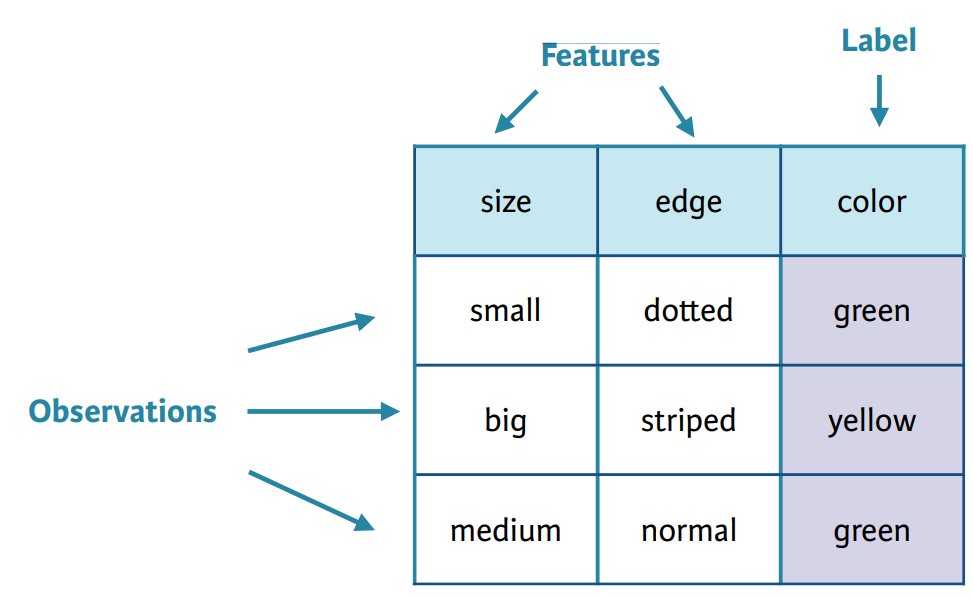# ML Building Blocks: Services and Terminology

## Stages

1. Training
• Refers to how machine uses historical data sets to build its prediction algorithms.
2. Model
• Model is what your machine creates after it’s been trained and refines over time as it learns.
3. Prediction
• Prediction is machine’s best estimate of what the outcome of specific input or set of inputs would be. It’s sometimes called the Inference of a Model.

## Data

In Training Process, Data is split into:

• Training Dataset
• Used by machine to create first model. Constitutes the majority of data.
• Test Dataset
• Is used to test the model for accuracy.

## Process / ML Workflow

Goal of Machine Learning model is to provide solution to a Business Problem. This happens through prediction. Prediction is not accurate and improves over time through provided feedback.

### ML Problem Framing

• Forming Machine Learning Problem from the Business Problem
• What to use and how to use it?
• Do we have all the data needed?
• Supervised Learning
• Learning from historical data set with a known answer.
• Unsupervised Learning
• Outcome is not known, ML algorithm will choose how to quantify the data and then give us the result.
• Reinforcement Learning
• The algorithm is rewarded based on the choices it makes while learning.

Classification Problems

• Binary Classification
• 2 classes
• Multiclass Classification
• 3 + classes

Problem Definition

• Defining:
• Observations
• Labels (Variables we are trying to predict)
• Features (Feature Engineering Process)### Data Collection / Integration

• Structured data
• Semi-structured data
• Unstructured

### Data Preparation

#### Data Cleaning

• Handling outliers
• Handing missing feature values
• Introduce new indicator variable to represent missing value
• Removes rows
• Imputation
• Replacing missing value with a value from dataset - may be a calculated guess. For example, for numerical we can use: mean, median.

#### Shuffling Training Data

Makes data order not important and improves the results in certain algorithms.

#### Test-Validation-Train Split

• Test: 20%
• Validation: 10%
• Train: 70%

#### Cross Validation

• Validation
• Leave-one-out (LOOCV)
• K-Fold

### Data Visualization & Analysis

Helps us understand the data better, refine the data, clean the outliers. This will result in better features leading to better models.

• Statistics
• Scatter-polts
• Could help detect feature correlations
• Histograms
• Will help us detect outliers and skews in data

### Feature Engineering

Process of converting raw data into more useful features.

• Numeric Value Binning
• Helps introduce non-linearity into linear models, breaking up continuous values
• Continuous values can be partioned into Bins based on ranges
• Deriving new non-linear features by combining feature pairs
• Non-Linear Feature Transformations
• Tree Path Features
• Uses leaves of decision tree as features
• Domain-Specific Transformations
• Text: stop words removal / stemming, lowercasing, puctuation, cutting off very high / low percentiles
• Web-page Features: multiple fields of text, URL, anchor text, relative style and positioning

### Model Training

Parameters are the knobs used to tune our Machine Learning Algorithm.

#### Parameter Turning

• Loss Function
• Predicts how far your predictions are from the ground truth values.
• Mean Square Loss
• Hinge Loss
• Logistic Loss
• Regularization
• Prevent overfitting by constraining weights to be small.
• Learning Parameters
• How fast or slow will your algorithm learn. Learning too fast may mean the algorithm will never reach the optimum value. Learning too slow means algorithm may take too long and never converge to the optimum.

### Model Evaluation

• Overfitting & Underfitting
• Evaluation Metrics (Will be checked on test dataset.)
• Regression
• Root Mean Square Error (RMSE)
• MAPE (Mean Absolute Percent Error)
• $R^2$
• Classification
• Confusion Matrix
• ROC Curve
• Precision-Recall

• How well the model is performing related to business goals
• Make the decision to deploy or not
• Accuracy
• Model generalization on unseen/unknown data